### 福特(进口) 福克斯(进口)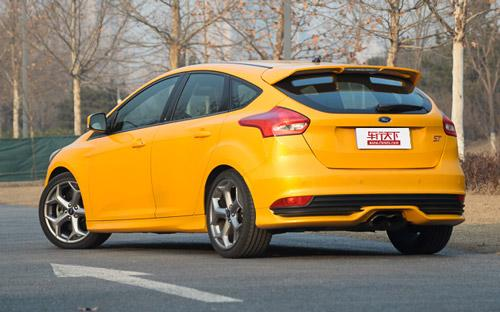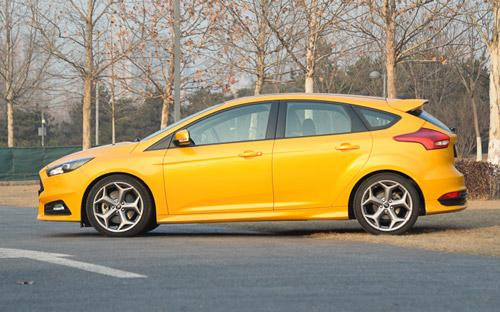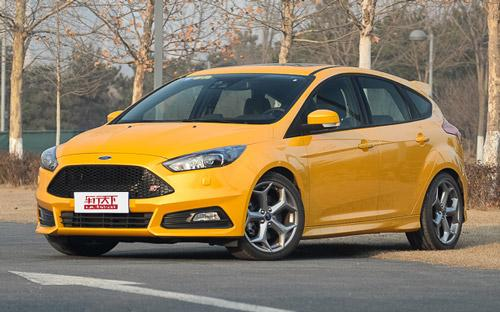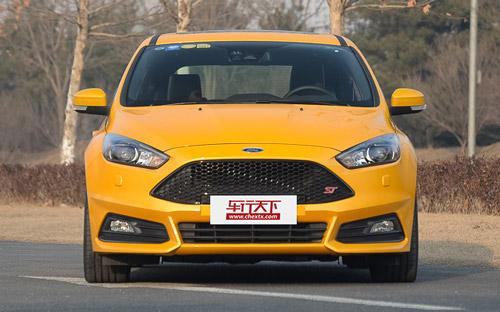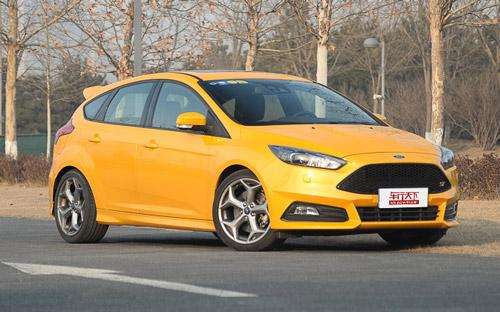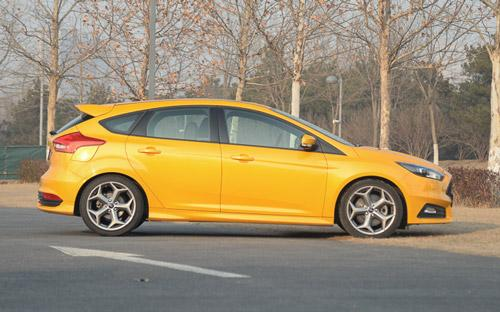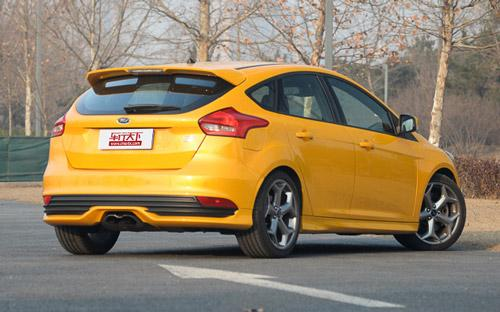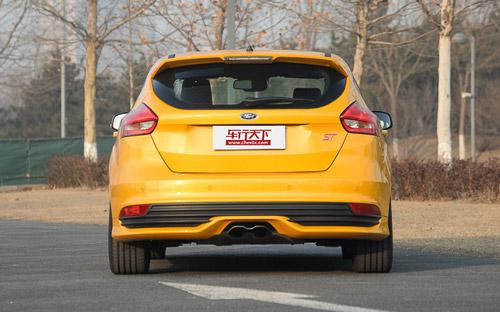4 种颜色可选2015款最低售价：25.98 万元起

4362(mm)1823(mm)1484(mm)##### 配置亮点：
• 胎压监测装置

• ISOFIX儿童座椅接口

• 车身稳定控制(ESC/ESP/DSC等)

• 电动天窗

• 定速巡航

• 后倒车雷达

• 真皮座椅

• GPS导航系统

• 氙气大灯

• 后视镜加热

• 提交
2015款 2.0T ST 橙色版 (267张)
• 2015款 2.0T ST 橙色版 (267张)
• 2015款 2.0T ST 标准版 (143张)
• 福特(进口) 福克斯(进口) 绕车实拍• 福特(进口) 福克斯(进口) 在售车型

排量 车型 厂商指导价 本地最低报价 购车工具
2.0T
2.0T ST 标准版 6挡手动
25.98万
25.98万

2.0T ST 橙色版 6挡手动
26.58万
26.58万

福特(进口) 福克斯(进口) 经销商

查看更多 >>

### 福特(进口) 福克斯(进口) 动力加速

福克斯(进口) 0-100公里加速时间分布在 6.5-6.5秒 属于 高性能级

动力级别 加速时间 车型
高性能级(1款)
6.5s
2.0T ST 标准版2.0T ST 橙色版

福特(进口) 福克斯(进口) 视频

福特(进口) 福克斯(进口) 新闻资讯

# 20万元买什么汽车最符合自己 买好车为你支招

汽车导购 超过8638次关注

20万左右买什么车？往往千挑万选却越发眼花缭乱。今天，汽车电商买好车为你支招，让你尽享20万车型的轻奢主义。在买好车电商平台购车，不仅可以享受难得的价格优惠...

# 新福克斯RS领衔 福特将推12款高性能车

进口新车 超过6467次关注

日前，福特宣布未来将会推出至少12款高性能车型，在这些车中包括了全新一代福克斯RS。

# 增柴油版选择 福特改款福克斯ST新消息

车系文章 超过3217次关注

在改款福克斯亮相日内瓦车展之后，近日福特高层透露了其高性能衍生版本ST的新信息，据悉，改款福克斯ST将会增加柴油动力选择，预计该车型0-100km/h加速时间在8秒。

# 融入轿跑车元素 福特研发新一代福克斯

车系文章 超过3120次关注

日前，海外媒体报道，福特正在开发下一代福克斯车型，未来该车外形将加入轿跑车的设计元素。

# 越来越有型 新福克斯北京车展静态评测

车系文章 超过4220次关注

没进展馆前就听说新一代的福克斯会亮相北京车展，这让很多人都特别期待，位于W2馆最里面的福特展台，一个需要爬楼梯才能上去的地方，新福克斯就高调的停在上面，周...

# 蜕变进行时 车展实拍新福克斯两厢版

车系文章 超过4070次关注

在2014日内瓦车展上，福特汽车旗下的新款福克斯两厢版车型与公众见面。新车在车头处出现了明显的变化，即前脸采用了全新的家族式“马丁”脸谱，而在车尾却仍旧保持...

# 2014纽约车展：福特新款福克斯三厢版

车系文章 超过3272次关注

纽约车展正式开幕，福特在本次车展上发布了新款福克斯三厢版的实车。新车相比现款车型主要针对外观进行了一些调整。

# 深化运动理念 福特新款福克斯车展

车系文章 超过3121次关注

在本届纽约车展中，福特为我们带来了新款福克斯的三厢版车型。与较早发布的两厢版相比，三厢车型的变化可谓是如出一辙。家族化的前脸设计有效地提升了豪华氛围，而...

猜你喜欢

﻿
• 快速找车
• 选择品牌
• 选择品牌
• A  奥迪
• A  阿斯顿·马丁
• A  阿尔法·罗密欧
• B  宝沃
• B  布加迪
• B  巴博斯
• B  保时捷
• B  宾利
• B  奔驰
• B  宝马
• B  本田
• B  别克
• B  标致
• B  比亚迪
• B  宝骏
• B  北汽制造
• B  北汽新能源
• B  北汽幻速
• B  北汽威旺
• B  北京汽车
• B  奔腾
• B  北汽绅宝
• C  长安
• C  长安商用
• C  长城
• C  昌河
• D  大众
• D  道奇
• D  DS
• D  东南
• D  东风风神
• D  东风风行
• D  东风小康
• D  东风风度
• D  东风
• F  福特
• F  丰田
• F  菲亚特
• F  法拉利
• F  福田
• F  福迪
• F  福汽启腾
• G  观致
• G  广汽传祺
• G  广汽吉奥
• G  GMC
• H  红旗
• H  汉腾汽车
• H  哈弗
• H  哈飞
• H  海格
• H  海马
• H  华颂
• H  黄海
• H  华泰
• H  恒天
• J  吉利汽车
• J  捷豹
• J  Jeep
• J  江淮
• J  江铃
• J  金杯
• J  九龙
• J  金旅
• K  凯翼
• K  凯迪拉克
• K  克莱斯勒
• K  科尼塞克
• K  卡威
• K  开瑞
• L  路虎
• L  林肯
• L  劳斯莱斯
• L  兰博基尼
• L  雷克萨斯
• L  铃木
• L  雷诺
• L  理念
• L  力帆
• L  莲花汽车
• L  猎豹
• L  路特斯
• L  陆风
• M  马自达
• M  MG
• M  MINI
• M  玛莎拉蒂
• M  摩根
• M  迈凯轮
• N  纳智捷
• O  欧宝
• O  讴歌
• O  欧朗
• Q  奇瑞
• Q  起亚
• Q  启辰
• R  日产
• R  荣威
• R  瑞麒
• S  三菱
• S  斯威汽车
• S  萨博
• S  smart
• S  斯柯达
• S  斯巴鲁
• S  思铭
• S  双龙
• S  上汽大通
• S  双环
• T  特斯拉
• T  腾势
• W  沃尔沃
• W  五菱汽车
• W  五十铃
• W  威兹曼
• W  威麟
• X  现代
• X  雪佛兰
• X  雪铁龙
• X  西雅特
• Y  一汽
• Y  英菲尼迪
• Y  英致
• Y  依维柯
• Y  野马汽车
• Y  永源
• Z  众泰
• Z  中华
• Z  中兴
• Z  知豆
• 选择车系
• 选择车系
• 车型对比
• 选择品牌
• 选择品牌
• A  奥迪
• A  阿斯顿·马丁
• A  阿尔法·罗密欧
• B  宝沃
• B  布加迪
• B  巴博斯
• B  保时捷
• B  宾利
• B  奔驰
• B  宝马
• B  本田
• B  别克
• B  标致
• B  比亚迪
• B  宝骏
• B  北汽制造
• B  北汽新能源
• B  北汽幻速
• B  北汽威旺
• B  北京汽车
• B  奔腾
• B  北汽绅宝
• C  长安
• C  长安商用
• C  长城
• C  昌河
• D  大众
• D  道奇
• D  DS
• D  东南
• D  东风风神
• D  东风风行
• D  东风小康
• D  东风风度
• D  东风
• F  福特
• F  丰田
• F  菲亚特
• F  法拉利
• F  福田
• F  福迪
• F  福汽启腾
• G  观致
• G  广汽传祺
• G  广汽吉奥
• G  GMC
• H  红旗
• H  汉腾汽车
• H  哈弗
• H  哈飞
• H  海格
• H  海马
• H  华颂
• H  黄海
• H  华泰
• H  恒天
• J  吉利汽车
• J  捷豹
• J  Jeep
• J  江淮
• J  江铃
• J  金杯
• J  九龙
• J  金旅
• K  凯翼
• K  凯迪拉克
• K  克莱斯勒
• K  科尼塞克
• K  卡威
• K  开瑞
• L  路虎
• L  林肯
• L  劳斯莱斯
• L  兰博基尼
• L  雷克萨斯
• L  铃木
• L  雷诺
• L  理念
• L  力帆
• L  莲花汽车
• L  猎豹
• L  路特斯
• L  陆风
• M  马自达
• M  MG
• M  MINI
• M  玛莎拉蒂
• M  摩根
• M  迈凯轮
• N  纳智捷
• O  欧宝
• O  讴歌
• O  欧朗
• Q  奇瑞
• Q  起亚
• Q  启辰
• R  日产
• R  荣威
• R  瑞麒
• S  三菱
• S  斯威汽车
• S  萨博
• S  smart
• S  斯柯达
• S  斯巴鲁
• S  思铭
• S  双龙
• S  上汽大通
• S  双环
• T  特斯拉
• T  腾势
• W  沃尔沃
• W  五菱汽车
• W  五十铃
• W  威兹曼
• W  威麟
• X  现代
• X  雪佛兰
• X  雪铁龙
• X  西雅特
• Y  一汽
• Y  英菲尼迪
• Y  英致
• Y  依维柯
• Y  野马汽车
• Y  永源
• Z  众泰
• Z  中华
• Z  中兴
• Z  知豆
• 选择车系
• 选择车系
• 选择车型
• 选择车型
• 意见反馈